# Build a Fraud Detection model on Cloud AI Platform with TensorFlow Enterprise and BigQuery

In this lab, you'll directly ingest a BigQuery dataset and train a fraud detection model with TensorFlow Enterprise on Google Cloud AI Platform.

## What you learn

You'll learn how to:

• Analyze data on BigQuery
• Ingest data using the BigQuery connector in TensorFlow Enterprise
• Build a deep learning model to detect fraud with an imbalanced dataset

You'll need a Google Cloud Platform project with billing enabled to run this codelab. To create a project, follow the instructions here.

## Step 1: Access the BigQuery public dataset

Follow this link to access BigQuery public datasets in the Google Cloud console.

In the Resources Tree in the bottom-left corner, you will see a list of datasets. Navigate through the available datasets until you find ml-datasets, and then select the ulb-fraud-detection table within it: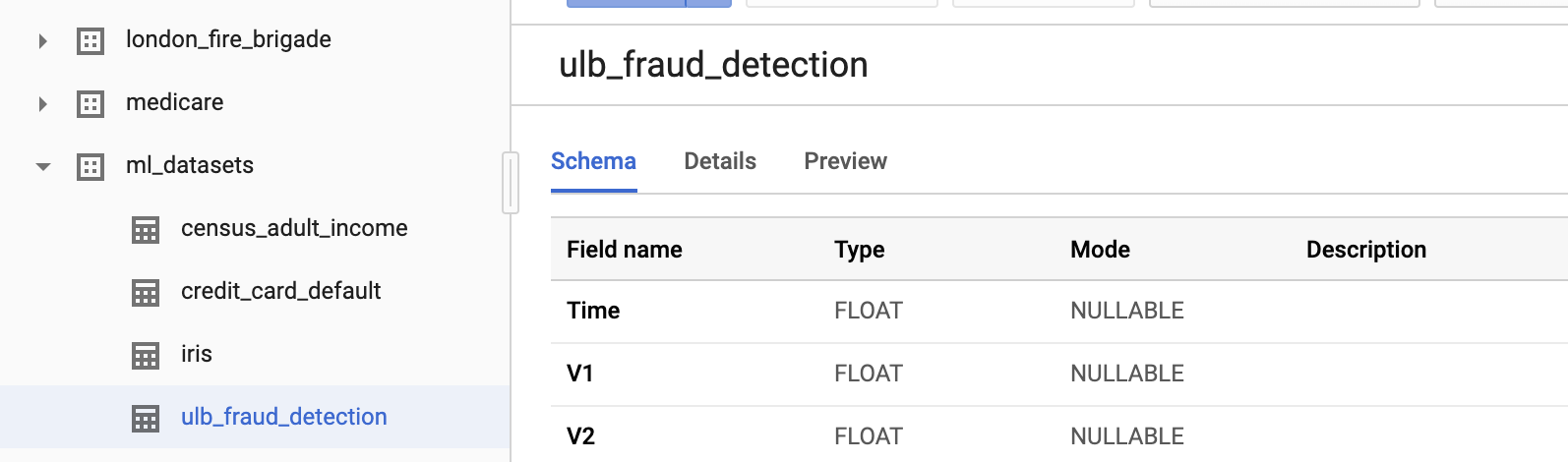Click around each tab to find out more about the dataset:

• The Schema tab describes the data types.
• The Details tab explains that this is an unbalanced dataset with 284,407 transactions, of which 492 are fraudulent.
• The Preview tab shows records from the dataset.

## Step 2: Query the Table

The details tab tells us this about the data:

• Time is the number of seconds between the first transaction in the dataset and the time of the selected transaction.
• V1-V28 are columns that have been transformed via a dimensionality reduction technique called PCA that has anonymized the data.
• Amount is the transaction amount.

Let's take a closer look by clicking Query Table to run a query:Update the statement to add a * to view all columns, and click Run.

``````SELECT * FROM `bigquery-public-data.ml_datasets.ulb_fraud_detection` LIMIT 1000
``````

## Step 3: Analyze the Data

BigQuery provides a number of statistical functions. Let's take a peek at how the data correlates with the target variable Class.

``````SELECT CORR(Time,Class) as TimeCorr, CORR(V1,Class) as V1Corr, CORR(V2,Class) as V2Corr, CORR(Amount,Class) as AmountCorr FROM `bigquery-public-data.ml_datasets.ulb_fraud_detection`
``````Correlation will provide a range from -1 (negatively correlated) to 1 (positively correlated), with 0 being independent.

Note that V1 and V2 have slight correlation with our target variable (around -0.1 and .1 respectively).

We don't see much correlation with Time. A slightly negatively correlation might tell us that there are fewer fraudulent transactions over time in the dataset.

Amount has even a lower correlation, indicating that fraudulent transactions are very slightly more likely in higher transaction amounts.

Step 4: Calculate mean values for feature scaling

Normalizing feature values can help a neural network converge faster. A common scheme is to center values around 0 with a standard deviation of 1. The following query will retrieve the mean values. Saving the result is not required, as we'll have a code snippet for that later.

You will also notice that the query includes an interesting WHERE clause. We'll describe that in the next section, when we cover how to split the data between training and test sets.

``````SELECT
AVG(Time), AVG(V1), AVG(V2), AVG(V3), AVG(V4), AVG(V5), AVG(V6), AVG(V7), AVG(V8),
AVG(V9), AVG(V10),AVG(V11), AVG(V12), AVG(V13), AVG(V14), AVG(V15), AVG(V16),
AVG(V17), AVG(V18), AVG(V19), AVG(V20), AVG(V21), AVG(V22), AVG(V23), AVG(V24),
AVG(V25), AVG(V26), AVG(V27),AVG(V28), AVG(Amount)
FROM
`bigquery-public-data.ml_datasets.ulb_fraud_detection`
WHERE
MOD(ABS(FARM_FINGERPRINT(CONCAT(SAFE_CAST(Time AS STRING),
SAFE_CAST(Amount AS STRING)))),10) < 8
``````

Step 5: Splitting the data

It's common practice to use 3 data sets when building a machine-learning model:

• Training: used to build the model by iteratively adjusting parameters
• Validation: used to assess if the model is overfitting by verifying on independent data during the training process
• Test: used after the model has been created to assess accuracy

In this codelab, we will use an 80/10/10 train/validation/test split.

We will put each dataset into its own table in BigQuery. The first step is to create a BigQuery "dataset" - which is a container for related tables. With your project selected, select Create Dataset.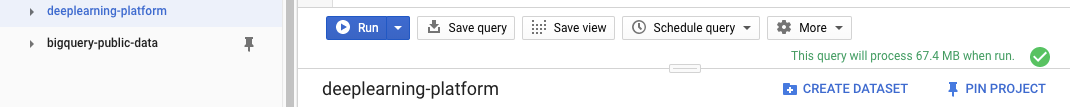Then, create a dataset called tfe_codelab to contain the train, validation, and test tables.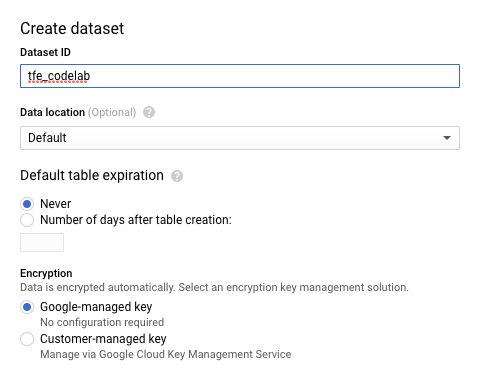Now, we will run 3 queries for train, test, and validation and save the data within the new tfe_codelab dataset.

In the Query editor, run a query to generate the training data:

``````SELECT *
FROM `bigquery-public-data.ml_datasets.ulb_fraud_detection`
WHERE MOD(ABS(FARM_FINGERPRINT(CONCAT(SAFE_CAST(Time AS STRING),SAFE_CAST(Amount AS STRING)))),10) < 8
``````

When the query has completed, save the results into a BigQuery table.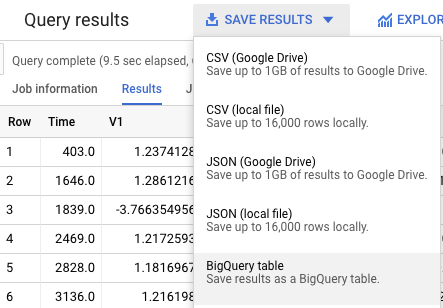Within the tfe_codelab dataset you just created, name the table ulb_fraud_detection_train and save the data.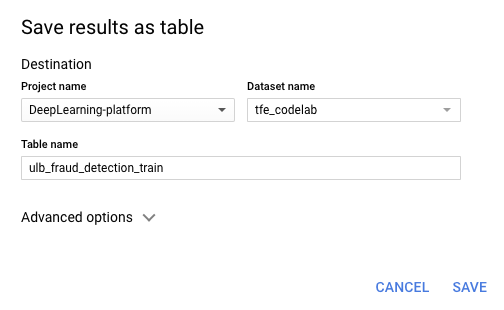The WHERE clause first splits the data by computing a hash on a couple columns. Then, it selects rows where the remainder of the hash when divided by 10 is below 80, giving us 80%.

Let's now repeat the same process for validation and test sets with similar queries that select 10% of the data each.

### Validation

``````SELECT *
FROM `bigquery-public-data.ml_datasets.ulb_fraud_detection`
WHERE MOD(ABS(FARM_FINGERPRINT(CONCAT(SAFE_CAST(Time AS STRING),SAFE_CAST(Amount AS STRING)))),10) = 8
``````

Save the results of this query into a table called ulb_fraud_detection_val.

### Test

``````SELECT *
FROM `bigquery-public-data.ml_datasets.ulb_fraud_detection`
WHERE MOD(ABS(FARM_FINGERPRINT(CONCAT(SAFE_CAST(Time AS STRING),SAFE_CAST(Amount AS STRING)))),10) = 9
``````

Save the results of this query into a table called ulb_fraud_detection_test.

## Step 1: Enable APIs

The BigQuery connector uses the BigQuery Storage API. Search for the BigQuery Storage API in the console and enable the API if it is currently disabled.## Step 2: Create an AI Platform Notebooks instance

Navigate to AI Platform Notebooks section of your Cloud Console and click New Instance. Then select the latest TensorFlow Enterprise 1.x instance type without GPUs:

##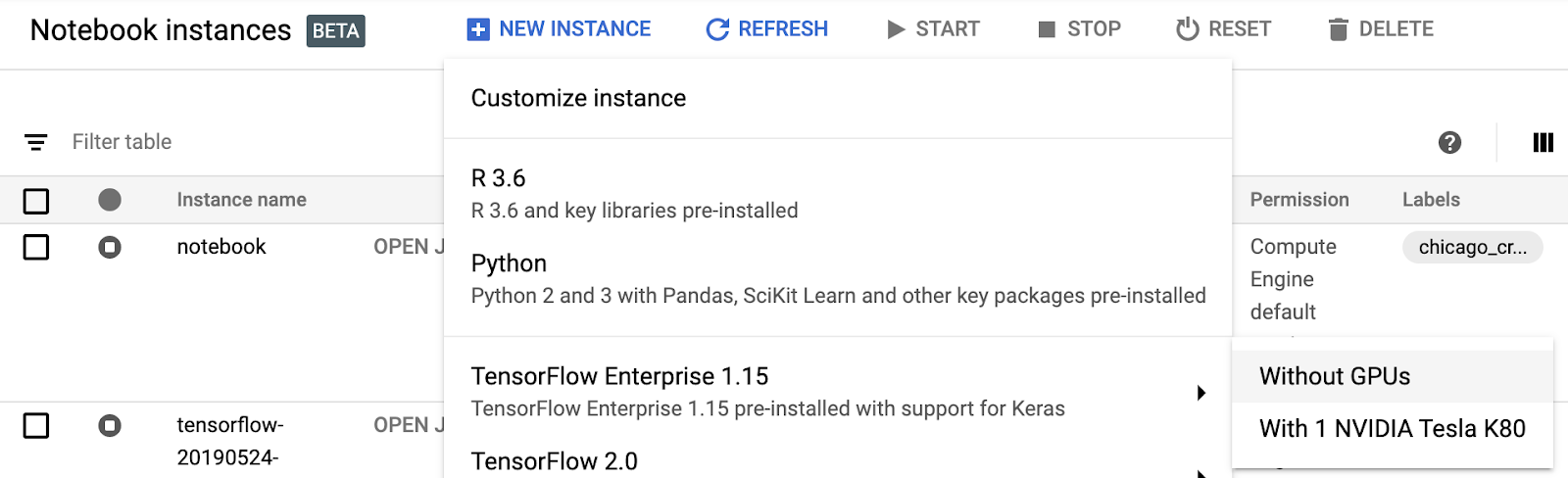Use the default options and then click Create. Once the instance has been created, select Open JupyterLab: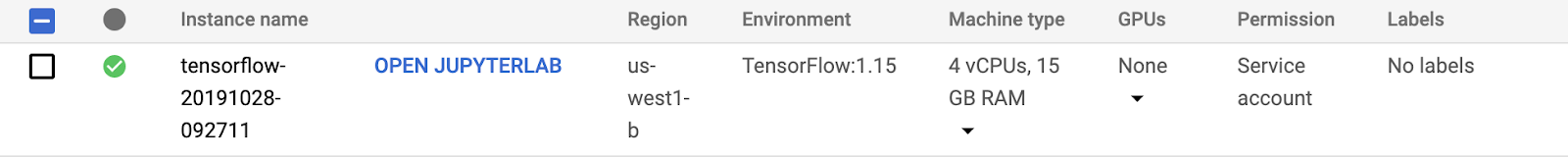Then, create a Python 3 notebook from JupyterLab: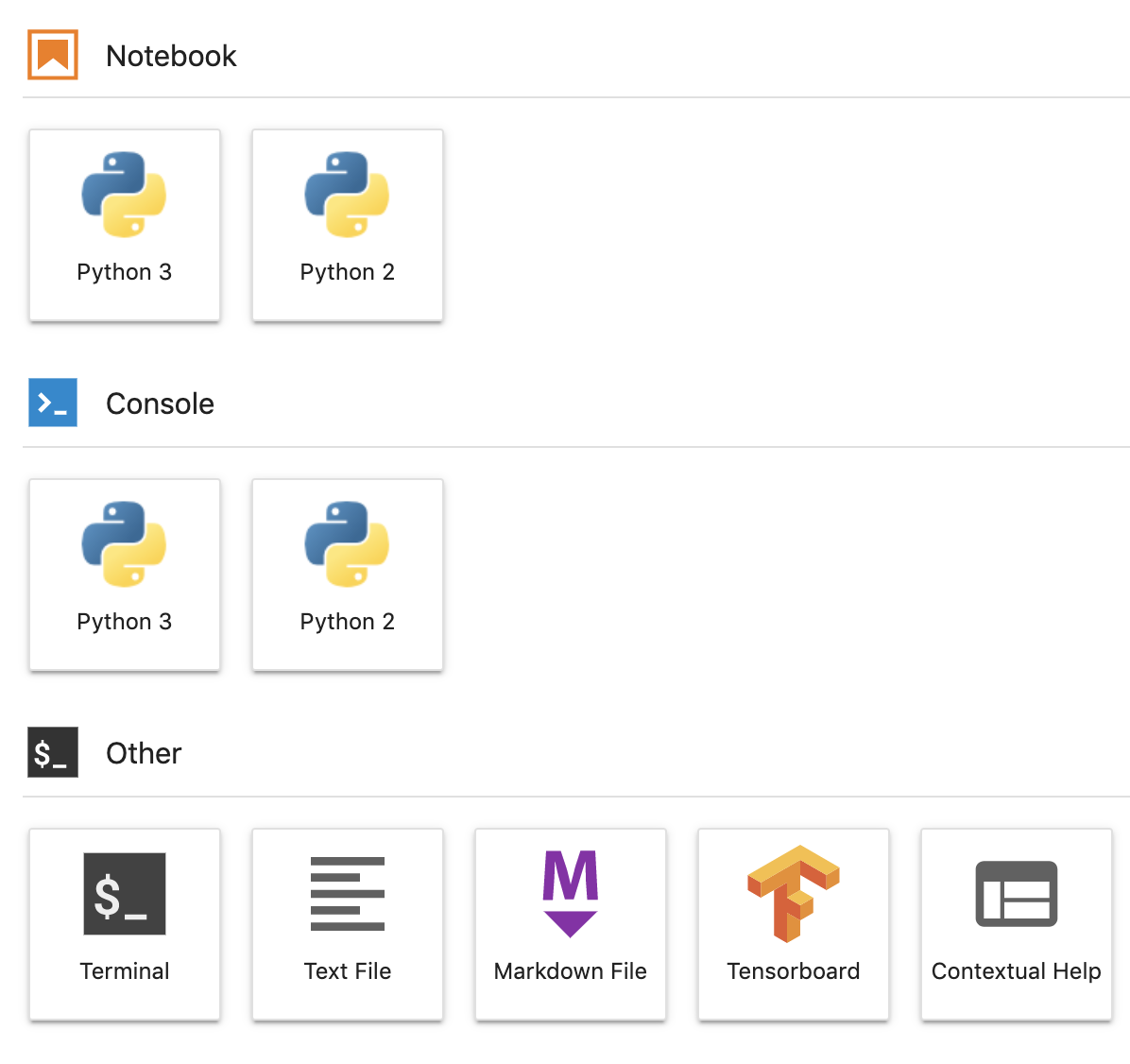## Step 1: Import Python packages

In the first cell of your notebook, add the following imports and run the cell. You can run it by pressing the right arrow button in the top menu or pressing command-enter:

``````import tensorflow as tf
import tensorflow.keras as keras
import tensorflow.keras.layers as layers

from tensorflow_io.bigquery import BigQueryClient

import functools

tf.enable_eager_execution()
``````

## Step 2: Define constants

Let's next define some constants for use in the project. Change GCP_PROJECT_ID to the actual project ID you are using. Go ahead and run new cells as you create them.

``````GCP_PROJECT_ID = '<YOUR_PROJECT_ID>'
DATASET_GCP_PROJECT_ID = GCP_PROJECT_ID # A copy of the data is saved in the user project
DATASET_ID = 'tfe_codelab'
TRAIN_TABLE_ID = 'ulb_fraud_detection_train'
VAL_TABLE_ID = 'ulb_fraud_detection_val'
TEST_TABLE_ID = 'ulb_fraud_detection_test'

FEATURES = ['Time','V1','V2','V3','V4','V5','V6','V7','V8','V9','V10','V11','V12','V13','V14','V15','V16','V17','V18','V19','V20','V21','V22','V23','V24','V25','V26','V27','V28','Amount']
LABEL='Class'
DTYPES=[tf.float64] * len(FEATURES) + [tf.int64]
``````

## Step 3: Define helper functions

Now, let's define a couple functions. read_session() reads data from a BigQuery table. extract_labels() is a helper function to separate the label column from the rest, so that the dataset is in the format expected by keras.model_fit() later on.

``````client = BigQueryClient()

def read_session(TABLE_ID):
return client.read_session(
"projects/" + GCP_PROJECT_ID, DATASET_GCP_PROJECT_ID, TABLE_ID, DATASET_ID,
FEATURES + [LABEL], DTYPES, requested_streams=2
)

def extract_labels(input_dict):
features = dict(input_dict)
label = tf.cast(features.pop(LABEL), tf.float64)
return (features, label)
``````

## Step 4: Ingest data

Finally, let's create each dataset and then print the first batch from the training dataset. Note that we have defined a BATCH_SIZE of 32. This is an important parameter that will impact the speed and accuracy of training.

``````BATCH_SIZE = 32

raw_train_data = read_session(TRAIN_TABLE_ID).parallel_read_rows().map(extract_labels).batch(BATCH_SIZE)
raw_val_data = read_session(VAL_TABLE_ID).parallel_read_rows().map(extract_labels).batch(BATCH_SIZE)
raw_test_data = read_session(TEST_TABLE_ID).parallel_read_rows().map(extract_labels).batch(BATCH_SIZE)

next(iter(raw_train_data)) # Print first batch
``````

## Step 1: Preprocess data

Let's create feature columns for each feature in the dataset. In this particular dataset, all of the columns are of type numeric_column, but there a number of other column types (e.g. categorical_column).

As we discussed earlier, we will also norm the data to center around zero so that the network converges faster. We've precalculated the means of each feature to use in this calculation.

``````MEANS = [94816.7387536405, 0.0011219465482001268, -0.0021445914636999603, -0.002317402958335562,
-0.002525792169927835, -0.002136576923287782, -3.7586818983702984, 8.135919975738768E-4,
-0.0015535579268265718, 0.001436137140461279, -0.0012193712736681508, -4.5364970422902533E-4,
-4.6175444671576083E-4, 9.92177789685366E-4, 0.002366229151475428, 6.710217226762278E-4,
0.0010325807119864225, 2.557260815835395E-4, -2.0804190062322664E-4, -5.057391100818653E-4,
-3.452114767842334E-6, 1.0145936326270006E-4, 3.839214074518535E-4, 2.2061197469126577E-4,
-1.5601580596677608E-4, -8.235017846415852E-4, -7.298316615408554E-4, -6.898459943652376E-5,
4.724125688297753E-5, 88.73235686453587]

def norm_data(mean, data):
data = tf.cast(data, tf.float32) * 1/(2*mean)
return tf.reshape(data, [-1, 1])

numeric_columns = []

for i, feature in enumerate(FEATURES):
num_col = tf.feature_column.numeric_column(feature, normalizer_fn=functools.partial(norm_data, MEANS[i]))
numeric_columns.append(num_col)

numeric_columns
``````

## Step 2: Build the model

Now we are ready to create a model. We will feed the columns we just created into the network. Then we will compile the model. We are including the Precision/Recall AUC metric, which is useful for imbalanced datasets.

``````model = keras.Sequential([
tf.keras.layers.DenseFeatures(numeric_columns),
layers.Dense(64, activation='relu'),
layers.Dense(64, activation='relu'),
layers.Dense(1, activation='sigmoid')
])

model.compile(loss='binary_crossentropy',
optimizer='adam',
metrics=['accuracy', tf.keras.metrics.AUC(curve='PR')])
``````

## Step 3: Train the model

There are a number of techniques to handle imbalanced data, including oversampling (generating new data in the minority class) and undersampling (reducing the data in the majority class).

For the purposes of this codelab, let's use a technique that overweights the loss when misclassifying the minority class. We'll specify a class_weight parameter when training and weight "1" (fraud) higher, since it is much less prevalent.

We will use 3 epochs (passes through the data) in this lab so training is quicker. In a real-world scenario, we'd want to run it long enough to the point where the stop seeing increases in accuracy of the validation set.

``````CLASS_WEIGHT = {
0: 1,
1: 100
}
EPOCHS = 3

train_data = raw_train_data.shuffle(10000)
val_data = raw_val_data
test_data = raw_test_data

model.fit(train_data, validation_data=val_data, class_weight=CLASS_WEIGHT, epochs=EPOCHS)
``````

## Step 4: Evaluate the model

The evaluate() function can be applied to test data that the model has never seen to provide an objective assessment. Fortunately, we've set aside test data just for that!

``````model.evaluate(test_data)
``````

## Step 5: Exploration

In this lab, we've demonstrated how to ingest a large data set from BigQuery directly into a TensorFlow Keras model. We've also walked through all the steps of building a model. Finally, we learned a bit about how to handle imbalanced classification problems.

Feel free to keep playing around with different architectures and approaches to the imbalanced dataset, to see if you can improve the accuracy!

If you'd like to continue using this notebook, it is recommended that you turn it off when not in use. From the Notebooks UI in your Cloud Console, select the notebook and then select Stop: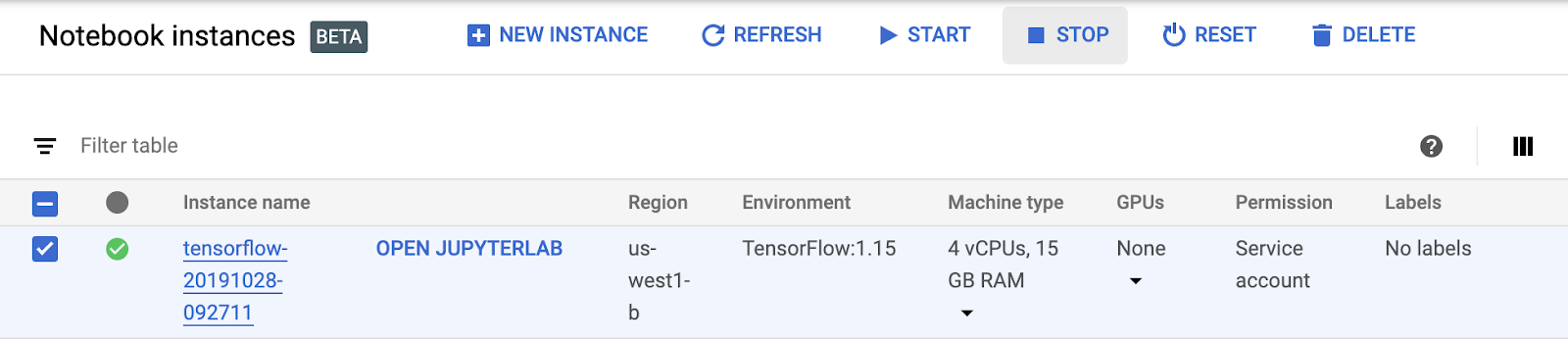If you'd like to delete all the resources you've created in this lab, simply Delete the notebook instance instead of stopping it.

Using the Navigation menu in your Cloud Console, browse to Storage and delete both buckets you created to store your model assets.